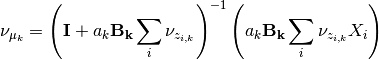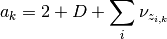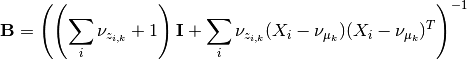This documentation is for scikit-learn version 0.15-gitOther versions

If you use the software, please consider citing scikit-learn.

# 2.1.3.2.1. Variational Gaussian Mixture Models¶

The API is identical to that of the GMM class, the main difference being that it offers access to precision matrices as well as covariance matrices.

The inference algorithm is the one from the following paper:

While this paper presents the parts of the inference algorithm that are concerned with the structure of the dirichlet process, it does not go into detail in the mixture modeling part, which can be just as complex, or even more. For this reason we present here a full derivation of the inference algorithm and all the update and lower-bound equations. If you’re not interested in learning how to derive similar algorithms yourself and you’re not interested in changing/debugging the implementation in the scikit this document is not for you.

The complexity of this implementation is linear in the number of mixture components and data points. With regards to the dimensionality, it is linear when using spherical or diag and quadratic/cubic when using tied or full. For spherical or diag it is O(n_states * n_points * dimension) and for tied or full it is O(n_states * n_points * dimension^2 + n_states * dimension^3) (it is necessary to invert the covariance/precision matrices and compute its determinant, hence the cubic term).

This implementation is expected to scale at least as well as EM for the mixture of Gaussians.

# 2.1.3.2.2. Update rules for VB inference¶

Here the full mathematical derivation of the Variational Bayes update rules for Gaussian Mixture Models is given. The main parameters of the model, defined for any class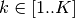are the class proportion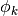, the mean parameters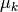, the covariance parameters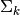, which is characterized by variational Wishart density,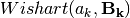, where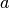is the degrees of freedom, and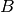is the scale matrix. Depending on the covariance parametrization,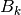can be a positive scalar, a positive vector or a Symmetric Positive Definite matrix.

## 2.1.3.2.2.1. The spherical model¶

The model then is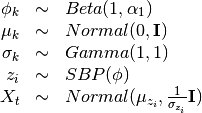The variational distribution we’ll use is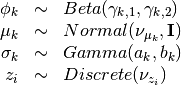### 2.1.3.2.2.1.1. The bound¶

The variational bound is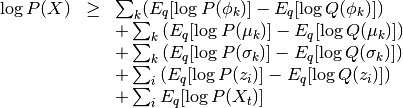The bound for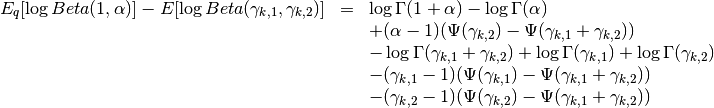The bound for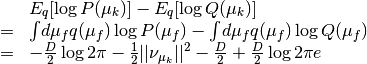The bound for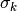Here I’ll use the inverse scale parametrization of the gamma distribution.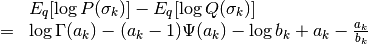The bound for z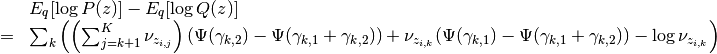The bound for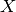Recall that there is no need for a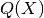so this bound is just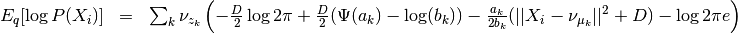For simplicity I’ll later call the term inside the parenthesis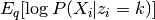Updating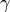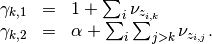Updating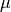The updates for mu essentially are just weighted expectations ofregularized by the prior. We can see this by taking the gradient of the bound with regards to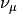and setting it to zero. The gradient is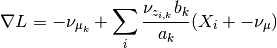so the update is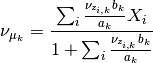Updatingand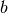For some odd reason it doesn’t really work when you derive the updates for a and b using the gradients of the lower bound (terms involving the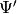function show up andis hard to isolate). However, we can use the other formula,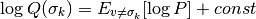All the terms not involvingget folded over into the constant and we get two terms: the prior and the probability of. This gives us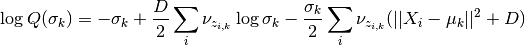This is the log of a gamma distribution, with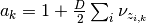and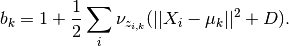You can verify this by normalizing the previous term.

Updating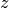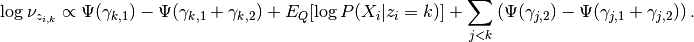## 2.1.3.2.2.2. The diagonal model¶

The model then is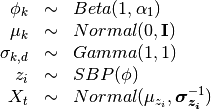Tha variational distribution we’ll use is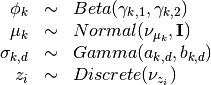### 2.1.3.2.2.2.1. The lower bound¶

The changes in this lower bound from the previous model are in the distributions of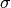(as there are a lot mores now) and.

The bound for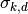is the same bound forand can be safely omitted.

The bound for:

The main difference here is that the precision matrix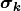scales the norm, so we have an extra term after computing the expectation of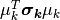, which is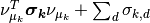. We then have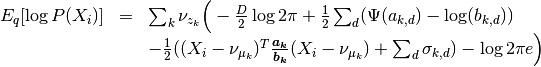The updates only chance for(to weight them with the new),(but the change is all folded into the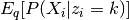term), and theandvariables themselves.

The update for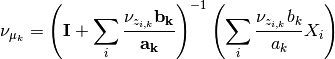The updates for a and b

Here we’ll do something very similar to the spheric model. The main difference is that now eachcontrols only one dimension of the bound: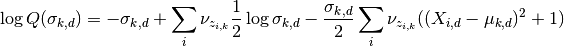Hence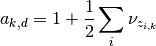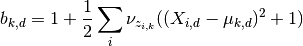## 2.1.3.2.2.3. The tied model¶

The model then is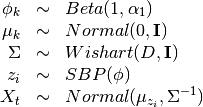Tha variational distribution we’ll use is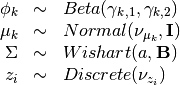### 2.1.3.2.2.3.1. The lower bound¶

There are two changes in the lower-bound: for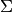and for.

The bound for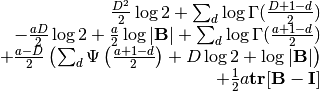The bound for X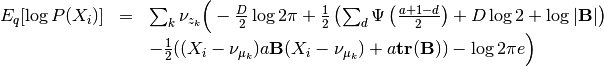As in the last setting, what changes are the trivial update for, the update forand the update forand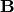.

The update for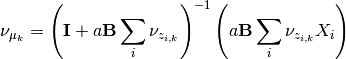The update forandAs this distribution is far too complicated I’m not even going to try going at it the gradient way.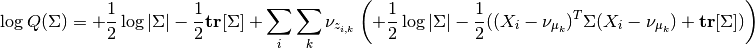which non-trivially (seeing that the quadratic form within the middle can be expressed as the trace of something) reduces to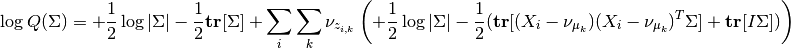hence this (with a bit of squinting) looks like a wishart with parameters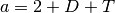and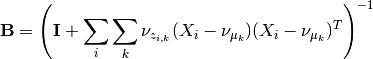## 2.1.3.2.2.4. The full model¶

The model then is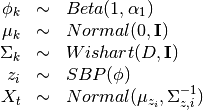The variational distribution we’ll use is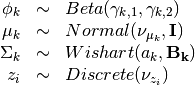### 2.1.3.2.2.4.1. The lower bound¶

All that changes in this lower bound in comparison to the previous one is that there are K priors on differentprecision matrices and there are the correct indices on the bound for X.